nextnano.com  nextnano³  Download | Search | Copyright | Publications  * password protected nextnano³ software2D TG MOSFET

# nextnano3 - Tutorial

## Electron wave functions of a 2D slice of a Triple Gate MOSFET

Author: Stefan Birner

`==> `This is the old website: A new version of this tutorial can be found here.

``` -> 2DSi_TGMOS_2Dcut_atGate_cl.in  -> 2DSi_TGMOS_2Dcut_atGate_qm.in  -> 2DSi_TGMOS_2Dcut_atGate_qm_iso.in  -> 3DSi_TGMOS_5nm_SD0V_G0V_qm.in  -> 3DSi_TGMOS_5nm_SD0V_G05V_qm.in```

## Triple Gate MOSFET (2D simulation)

A Triple Gate MOSFET is a nanowire if the dimensions along the x and y directions are only a few nanometers, thus quantization effects have to be taken into account.

The Si channel has a rectangular shape with a width of 5 nm and a height of 5 nm.

The Si channel is surrounded by SiO2 (thickness 1.5 nm).

The Si/SiO2 nanowire is surrounded by a Gate (at the left and right side, and at the top).

The following schematic shows a 2D slice of a 3D Triple Gate MOSFET.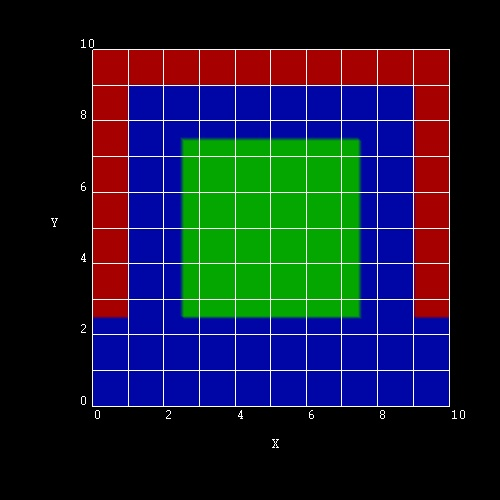In this tutorial we will only simulate this 2D slice and not the whole 3D structure.

We apply a voltage of 0.5 V to the Gates and solve the two-dimensional Schrödinger and Poisson equations self-consistently (including the SiO2 region).

There are six conduction band minima in silicon (Delta valleys).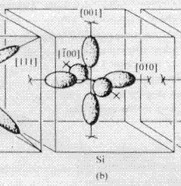Consequently, we have to solve three 2D Schrödinger equations with different effective mass tensor orientations because the mass tensor is not isotropic.

The longitudial  mass is 0.916 m0.
The transversal mass is 0.190 m0

Our Schrödinger equations are numbered` deg1`,` deg2`,``` deg3```.

`deg1: `a) mxx = mlongitudinal = 0.916 m0, myy = mtransversal = 0.190 m0
`deg2: `b) mxx = mtransversal = 0.190 m0, myy = mlongitudinal = 0.916 m0
`deg3: `c)  mxx = myy = mtransversal = 0.190 m0

The potential Ec(x,y) that enters the Schrödinger equation is the same in these three cases.
Note: The cases a) and b) are not identical (i.e. degenerate) because the potential is not symmetric with respect to exchanging x and y coordinates.

The following keyword and specifier can be used to output the effective mass tensors (1/mij). This is important to check how the Schrödinger equations are sorted with respect to their effective masses.
```  \$output-1-band-schroedinger    ...    effective-mass-tensor = yes```

### Electron wave functions (psi²)

`-> 2DSi_TGMOS_2Dcut_atGate_qm.in`

The lowest eigenstates for the cases a), b) and c) are the following:

`deg1: `a) mxx = mlongitudinal = 0.916 m0, myy = mtransversal = 0.190 m0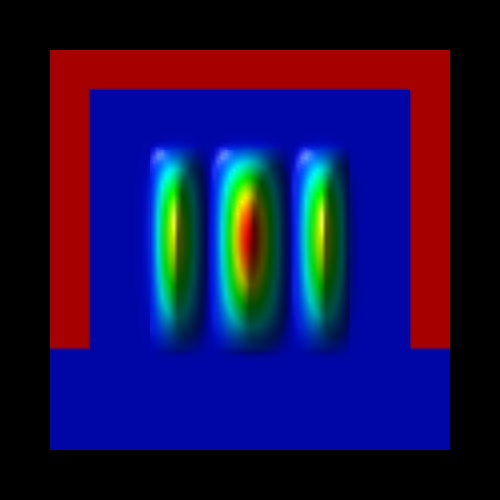`E1,deg1 = -26 meV` `E``2,deg1`` = -1 meV` `E``3,deg1`` = +77 meV`

Here, the heavier mass is along the y directon, and the lighter mass along the y direction.
The energy spacing between the two lowest subbands is about 25 meV.
The eigenvalues are contained in` Schroedinger_1band/ev2D_cb003_qc001_sg001_deg001_dir_Kx001_Ky001_Kz001.dat`.

`deg2: `b) mxx = mtransversal = 0.190 m0, myy = mlongitudinal = 0.916 m0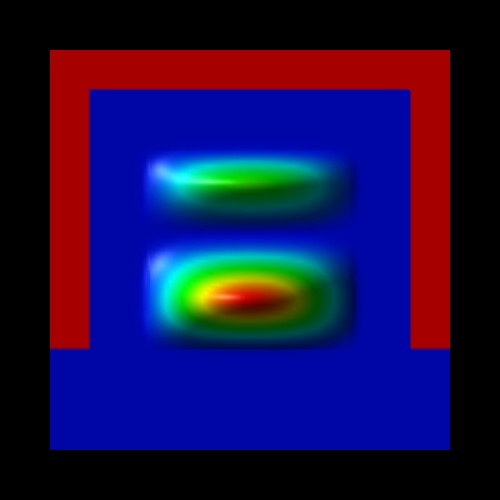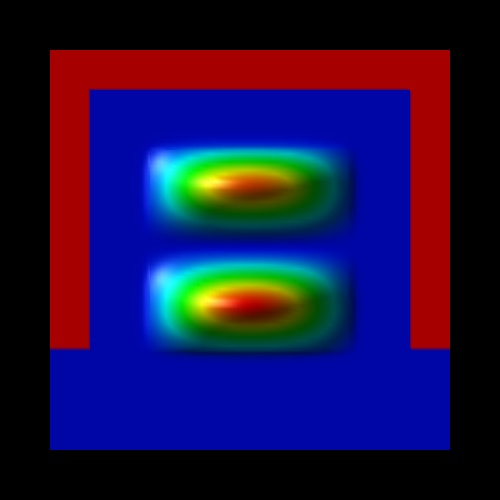`E1,deg2 = -28 meV` `E2,deg``2 = +6 meV` `E3,deg``2 = +82 meV`

Here, the lighter mass is along the x directon, and the heavier mass along the y direction.
The energy spacing between the two lowest subbands is about 35 meV.
The eigenvalues are contained in` Schroedinger_1band/ev2D_cb003_qc001_sg001_deg002_dir_Kx001_Ky001_Kz001.dat`.

`deg3: `c)  mxx = myy = mtransversal = 0.190 m0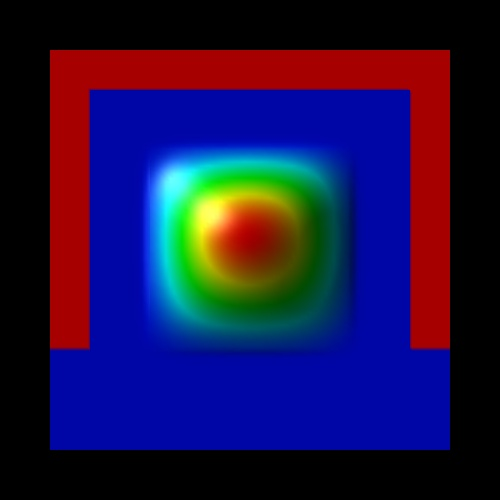`E1,deg``3 = +16 meV` `E2,deg``3 = +167 meV` `E3,deg``3 = +173 meV`

`E4,deg``3 = 323`,` ``E5,deg````3 = 462```,` ``E6,deg````3 = 470```,` ``E7,deg````3 = 615```,` ``E8,deg````3 = 616```

These eigenvalues have the lighter mass in the x and y directions. Consequently, their energies are much higher than in the other two Schrödinger equations.
The energy spacings between the lowest subbands is of the order 140-150 meV.
The eigenvalues are contained in` Schroedinger_1band/ev2D_cb003_qc001_sg001_deg003_dir_Kx001_Ky001_Kz001.dat`.
(Compare the wave functions and the energies with the isotropic case as discussed further below.)

### Electron density

The resulting electron density has the following shape:

The units are 1 x 1018 cm-3. The density has been calculated by occupying the eigenstates with respect to the Fermi level which is at 0 eV.
Note that the quantum mechanical density is close to zero near the Si/SiO2 interfaces because the wave functions tend to zero at the SiO2 barriers.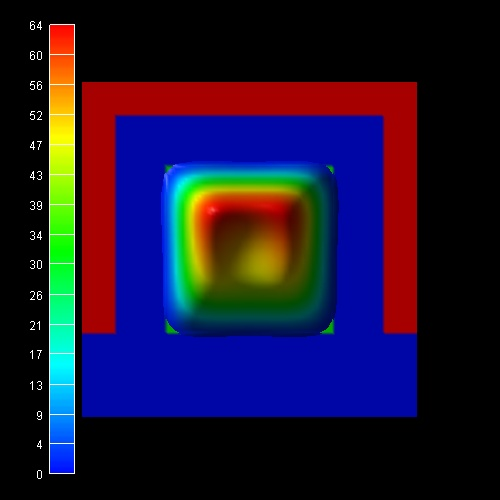The following figure shows the same quantum mechanical electron density together with two slices through the conduction band edges.
The units are in eV and the conduction band offset between SiO2 and Si is 3.1 eV.
At the gates, the conduction band edge is set to -0.5 eV, representing the applied bias of 0.5 eV.
One can clearly see that for silicon in the middle of the nanowire the conduction band has its highest value and its lowest value close at the Si/SiO2 interface.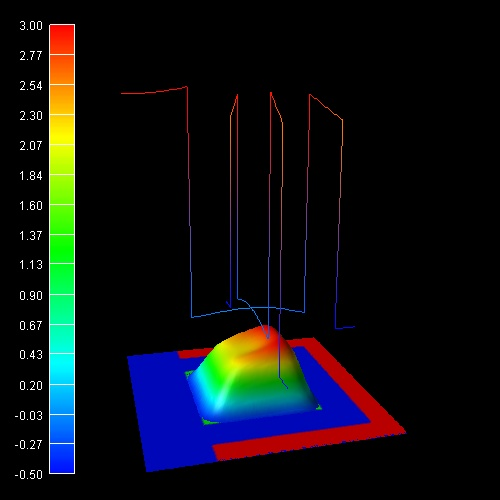If one had neglected the effect of quantum confinement, then the resulting classical electron density would have peaks near the Si/SiO2 interfaces as is shown in this figure.

`-> 2DSi_TGMOS_2Dcut_atGate_cl.in`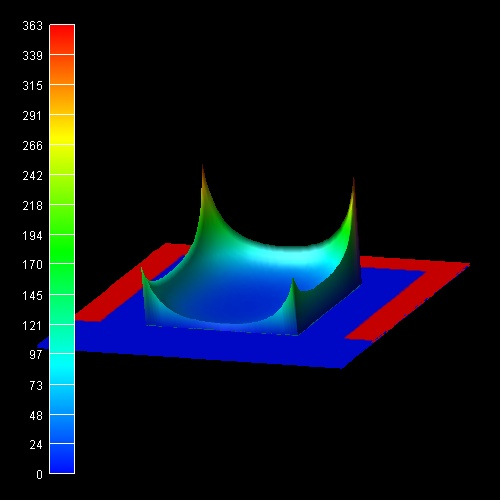Obviously, a realistic calculation of such transistors cannot be based on classical densities. The full 2D (or better 3D) Schrödinger equations have to be solved. The IV characteristics of such a quantum-mechanically calculated Triple Gate MOSFET transistor will be discussed in another tutorial.

### Isotropic electron masses

`-> 2DSi_TGMOS_2Dcut_atGate_qm_iso.in`

Very often, for simplicity, an isotropic electron mass for the Schrödinger equation is assumed.
E.g. the DOS (density of states) electron mass of Si in the Delta minima can be calculated as follows:

me*DOS = (ml·mt2)1/3 = (0.916·0.192)1/3 m0 = 0.321m0

More details...

In this case, only one Schrödinger equation has to be solved (in contrast to three equations as described above), thus` deg = 1`.

The wave functions and energies in this case are:

`deg1: `mxx = myy = mDOS = 0.321 m0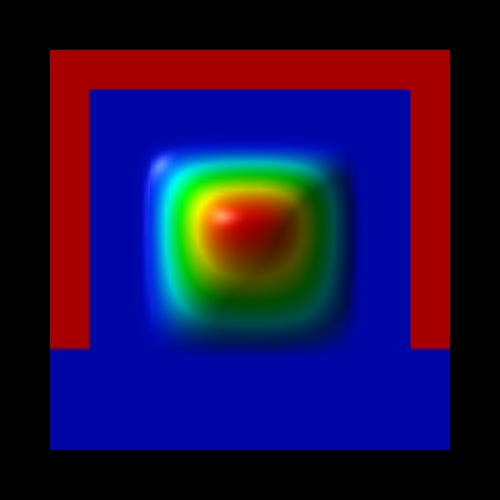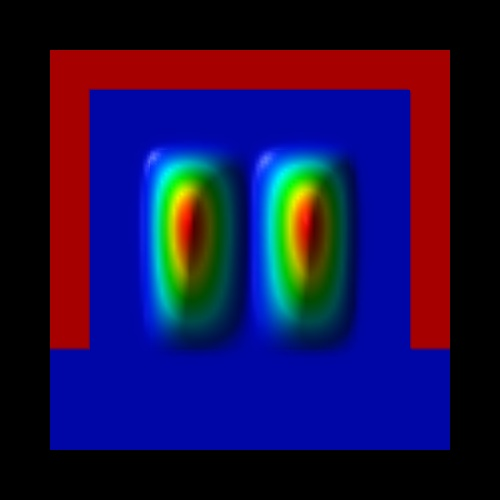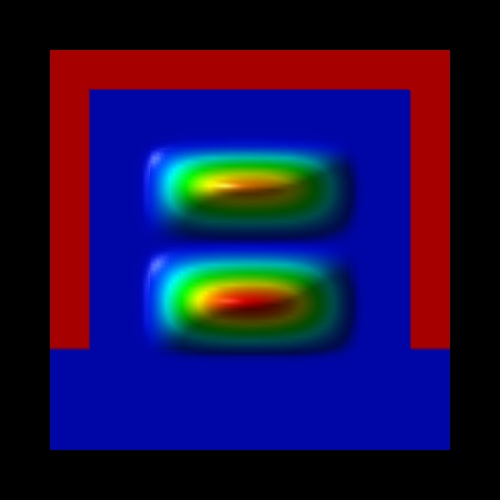`E1`` = -21 meV` `E2`` = +69 meV` `E3`` = +75 meV` `E``4 = +166 meV`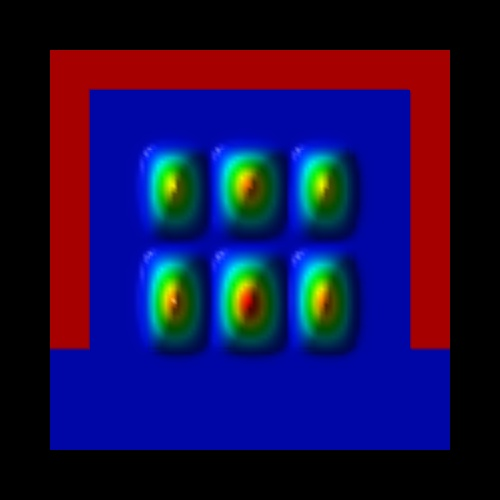`E``5 = +262 meV` `E6`` = +270 meV` `E7`` = +360 meV` `E``8 = +360 meV`

The wave functions (psi²) look very similar as in the case of` `"`deg3: ` c)"` `(see above) where the masses are isotropic in the (x,y) plane but here, the energy spacings between different subbands are smaller (around 90-100 meV) because the DOS mass is larger than the transversal masses (as in the case of` `"`deg3: `c)").

The eigenvalues are contained in` Schroedinger_1band/ev2D_cb003_qc001_sg001_deg001_dir_Kx001_Ky001_Kz001.dat`.

## 3D simulation of the Triple Gate MOSFET

``` -> 3DSi_TGMOS_5nm_SD0V_G0V_qm.in  -> 3DSi_TGMOS_5nm_SD0V_G05V_qm.in```

The following figures show the results of the self-consistent 3D Schrödinger-Poisson solution of this Triple Gate structure
(Si channel length = 25 nm, source region length = 10 nm, drain region length = 10 nm,
constant doping profile in source and drain region with a doping concentration of 1 * 1020 cm-3 (fully ionized)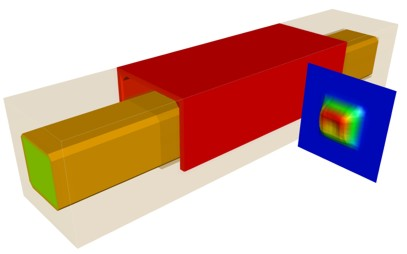Note: Compare this electron density with the density obtained in the 2D simulation as discussed above.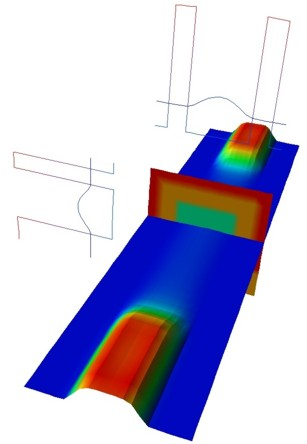closed channel VSD = 0.0 V VSG = 0.0 V open channel VSD = 0.0 V VSG = 0.5 V

The plots show the isosurfaces of the electron densities along 2D slices through the Triple Gate MOSFET. The lower two figures also show 1D slices of the conduction band profiles and 1D slices of the electron densities in the middle of the device.

The classical densities would look similar to the classical densities of the 2D calculations shown above.